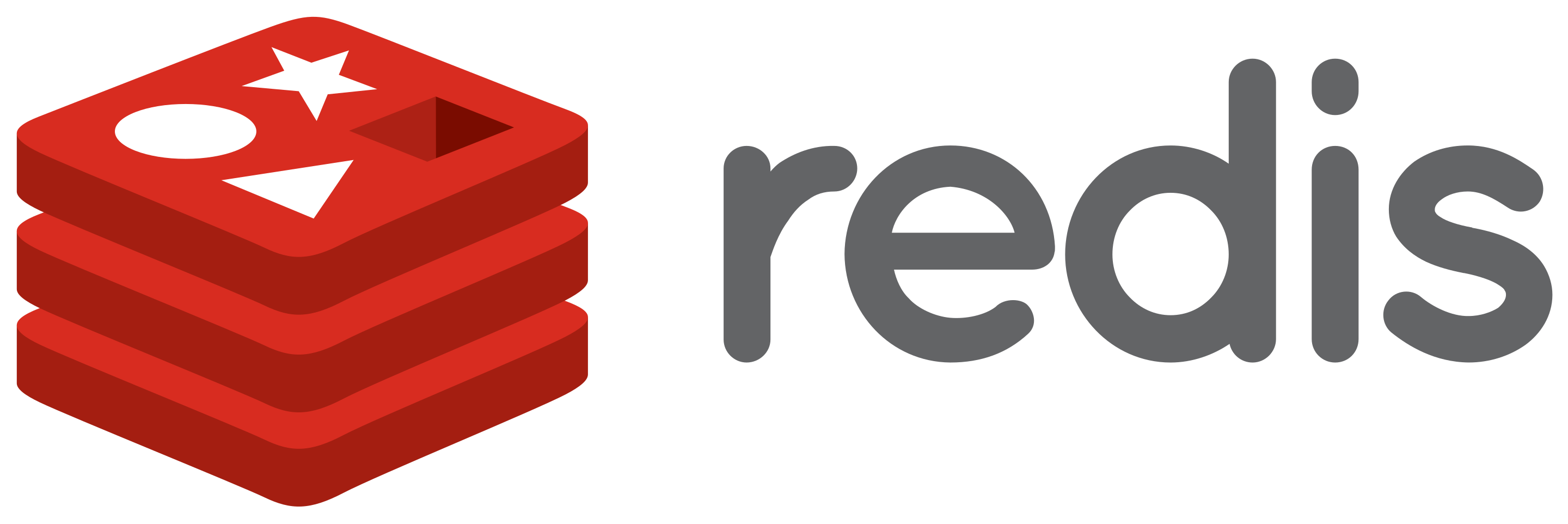# golang实现BST和AVL

AVL树得名于它的发明者 G. M. Adelson-Velsky和 E. M. Landis，他们在1962年的论文《An algorithm for the organization of information》中发表了它，它是最早的自平衡二分搜索树。# redis设计与实现系列1-SDS

redis自己构建的一种名为简单动态字符串（simple dynamic string，SDS）的抽象类型。# 常见的负载均衡算法# Docker快速部署一个属于你自己的博客

Docker快速部署一个solo博客# Array - 31. Next Permutation

Implement next permutation, which rearranges numbers into the lexicographically next greater permutation of numbers.# Array - 34. Find First and Last Position of Element in Sorted Array

Given an array of integers nums sorted in ascending order, find the starting and ending position of a given target value.# string- 43. Multiply Strings

Given two non-negative integers num1 and num2 represented as strings, return the product of num1 and num2, also represented as a string.# Array - 54. Spiral Matrix

Given a matrix of m x n elements (m rows, n columns), return all elements of the matrix in spiral order.# Array - 59. Spiral Matrix II

Given a positive integer n, generate a square matrix filled with elements from 1 to n2 in spiral order.# Binary Search - 378. Kth Smallest Element in a Sorted Matrix

Given a n x n matrix where each of the rows and columns are sorted in ascending order, find the kth smallest element in the matrix.# Array - 57. Insert Interval

Given a set of non-overlapping intervals, insert a new interval into the intervals (merge if necessary).# Array - 36. Valid Sudoku

Determine if a 9x9 Sudoku board is valid.# DFS&BFS - 37. Sudoku Solver

Write a program to solve a Sudoku puzzle by filling the empty cells.# String - 44. Wildcard Matching

Given an input string (s) and a pattern (p), implement wildcard pattern matching with support for '?' and '*'.# String - 67. Add Binary

Given two binary strings, return their sum (also a binary string).# String - 71. Simplify Path

Given an absolute path for a file (Unix-style), simplify it. Or in other words, convert it to the canonical path.# Spring事务的传播行为# Go modules 简介

Golang的版本管理视频推荐：Go with Versions - GopherConSG 2018# String - 8. String to Integer (atoi)

8.String to Integer (atoi) Implement atoi which converts a string to an integer. The function first discards as many whitespace characters as necessary until the first non-whitespace character is found. Then, starting from this character, takes an optional initial plus or minus sign followed by as many numerical digits as possible, and interprets them as a numerical value. The string can contain additional characters after those that form the integral number, which are ignored and have no effect....# Array - 16. 3Sum Closest

16. 3Sum Closest Given an array nums of n integers and an integer target, find three integers in nums such that the sum is closest to target. Return the sum of the three integers. You may assume that each input would have exactly one solution. Example: Given array nums = [-1, 2, 1, -4], and target = 1. The sum that is closest to the target is 2. (-1 + 2 + 1 = 2). 思路： 给定一个数组，找出三个数的和最接近target，和15题很像，做法类似，都是对于每一位元素，用双指针找出另外两个数，更新出一个最小的结果就可以。 代码： go： f....

Nothing just happens, it's all part of a plan.